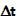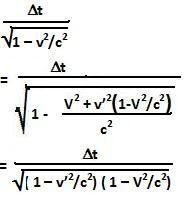## Sunday, November 22, 2009

### Irodov Problem 1.362In this problem we need to first determine what is the speed of the particle with respect to frame K.

For this problem we can use the solution we derived from Problem 1.358 namely,Here, we know that v'x = 0 (2a), v'y = v' (2b) and we need to find vx and vy.The magnitude of velocity of the particle as seen from frame K is given by,The particle will decay in timein its own frame. In frame K however, this will seem like a much longer time interval given by,The distance traveled by the particle during this time as seen by frame K is given by,#### 1 comment:Anonymous said...

i am in 11th trying for iit do i need to do irodov at this stage? because i cannot solve them so easily . your solutions are the only way i know how to solve the tougher sums but i can handle hc verma very well .